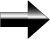Why A Zero In The Denominator Of A Fraction Is Undefined

Basically, if we have a zero in the denominator of a fraction, the value of the fraction becomes infinite.  We can never actually reach infinity.  Infinity is not a number, it is only a concept.

Here is an example to explain this:

Consider the following:  1000/1000 = 1, 1000/500 = 2, 1000/100 = 10, and 1000/10 = 100.  We can see here that as the denominator of the fraction becomes smaller, the value of the entire fraction becomes larger.  1000/0.001 = 1,000,000.  We can see that the value of the fraction increases extremely fast as the denominator approaches zero; and if the denominator actually gets to zero, the value of the fraction will have exploded to infinity, which is impossible (remember, infinity is only a concept and not an actual number, so it cannot be the value of our fraction).

Therefore, we can never have a zero in a denominator.  A zero in a denominator is undefined.

Main Pre-Algebra Page